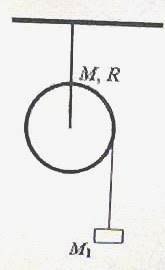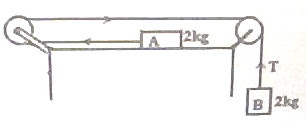## Pages

`“Life is like riding a bicycle.  To keep your balance you must keep moving.”–Albert Einstein`

## Wednesday, September 30, 2009

### AP Physics C – A Free Response Practice Question on Rotation

Essential points and some practice questions on rotational motion have been discussed on this site earlier. You can access all posts related to rotational motion on this site by clicking on the label ‘rotation’ below this post.

Today we will discuss a free response question (for practice) in this section. This

question is meant for AP Physics C aspirants:

A solid cylinder of mass M and radius R has a light inextensible string wound round it. The free end of the string is tied to a rigid support (Fig.) and the cylinder is released from its state of rest.

(a) Determine the acceleration ‘a of the cylinder as it moves down, unwinding the string.
The cylinder itself is now suspended from the support so that it is free to rotate about its axle. The friction at the axle of the cylinder is negligible. A mass M1 is now attached to the free end of the string wound round the cylinder as shown in the adjoining figure and the system is released from rest at time t = 0. Now answer the following questions (b), (c) and (d):(b) Determine the acceleration a1 of the mass M1 in terms of the given parameters.

(c) In terms of the given parameters obtain the angular acceleration of the cylinder when the mass M1 moves down, unwinding the string.

(d) Determine the kinetic energy of the cylinder at time t.

Try to answer this question which carries 15 points. You have 15 minutes at your disposal. I’ll be back with a model answer for you shortly.

Meanwhile find some useful multiple choice questions with solution in this section at physicsplus.

## Friday, September 11, 2009

### AP Physics B & C – Two Multiple Choice Questions (for practice) on Newton’s Laws of Motion

"Only two things are infinite, the universe and human stupidity, and I'm not sure about the former."
Albert Einstein

Two practice questions (MCQ) on Newton’s laws of motion are given below with solution. Even though these are meant for AP Physics C aspirants, they should not be difficult for those who are preparing for AP Physics B Exam.(1) In the system shown in the adjoining figure, the horizontal surface on which block A is placed is smooth and the pulleys are light and frictionless. If g = 10 ms–2, the tension T in the string (which may be assumed to be weightless and inextensible) is
(a) 1 N
(b) 2N
(c) 5 N
The velocity of the iron block at the moment of hitting the nail is 6 ms–1.
(d) 10 N
(e) 20 N
Net force driving the system = Weight of B = mg = 2×10 = 20 N.
Acceleration (a) of the system is given by
a = Driving force /Total mass moved = 20/4 = 5 ms–2.
Tension, T = Weight of B moving down (as in a lift) = m(ga) = 2(10 – 5) =10 N.(2) An iron block of mass 8 kg falling vertically down hits a nail on a block of wood (fig.). The velocity of the iron block at the moment of hitting the nail is 6 ms–1. If the nail penetrates through 2 cm into the wooden block due to the impact, what is the force exerted by the iron block on the nail?
(a) 1600 N
(b) 2200 N
(c) 4800 N
(d) 7200 N
(e) 9600 N
The acceleration (in fact, retardation), a of the iron block during the impact is given by
0 = 62 + 2a×(0.02), from the equation v2 = u2 + 2as
This gives a = – 900 ms–2
The negative sign shows that the iron block is retarded.
The retarding force exerted by the nail on the iron block is of magnitude F given by
F = ma = 8×900 = 7200 N
The force exerted by the iron block on the nail is equal and opposite to that exerted by the nail on the iron block. The answer therefore is 7200 N.
[The above question can be modified as follows:
An iron block of mass 8 kg falling freely from rest under gravity from a height of 1.8 m hits a nail on a block of wood (fig.). If the nail penetrates through 2 cm into the wooden block due to the impact, what is the force exerted by the iron block on the nail? (g = 10 ms–2)
(a) 1600 N
(b) 2200 N
(c) 4800 N
(d) 7200 N
(e) 9600 N
The answer will be unchanged since the velocity at the moment of hitting the nail will be 6 ms–1 itself (v = √(2gh) = √(2×10×1.8) = √(36) = 6)].